# Right triangle - math word problems

1. Forces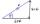Determine the resultant of two perpendicular forces F1 = 560 N and second force of 25% smaller.
2. CablewayCableway has a length of 1800 m. The horizontal distance between the upper and lower cable car station is 1600 m. Calculate how much meters altitude is higher upper station than the base station.
3. Broken treeThe tree was 35 meters high. The tree broke at a height of 10 m above the ground. Top but does not fall off it refuted on the ground. How far from the base of the tree lay its peak?
4. Journey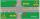Charles and Eva stands in front of his house, Charles went to school south at speed 5.4 km/h, Eva went to the store on a bicycle eastwards at speed 21.6 km/h. How far apart they are after 10 minutes?
5. Diamond ABCD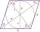In the diamond ABCD is the diagonal e = 24 cm and size of angle SAB is 28 degrees, where S is the intersection of the diagonals. Calculate the circumference of the diamond.
6. Construct 1Construct a triangle ABC, a = 7 cm, b = 9 cm with right angle at C, construct the axis of all three sides. Measure the length of side c (and write).
7. CarpetThe room is 10 x 5 meters. You have the role of carpet width of 1 meter. Make rectangular cut of roll that piece of carpet will be longest possible and it fit into the room. How long is a piece of carpet? Note .: carpet will not be parallel with the dia
8. Triangle ABCIn a triangle ABC with the side BC of length 2 cm The middle point of AB. Points L and M split AC side into three equal lines. KLM is isosceles triangle with a right angle at the point K. Determine the lengths of the sides AB, AC triangle ABC.
9. LiftThe largest angle at which the lift rises is 16°31'. Give climb angle in permille.
10. Ballistic curve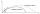The ballistic grenade was fired at a 45° angle. The first half ascended, the second fall. How far and how far it reached if his average speed was 1200km/h, and 12s took from the shot to impact.
11. Church tower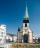Archdeacon church in Usti nad Labem has diverted tower by 186 cm. The tower is 65 m high. Calculate the angle by which the tower is tilted. Result write in degree's minutes.
12. 3sides prismThe base of vertical prism is an isosceles triangle whose base is 10 cm and the arm is 13 cm long. Prism height is three times the height of base triangle. Calculate the surface area of the prism.
13. Circular poolThe base of the pool is a circle with a radius r = 10 m, excluding a circular segment that determines the chord length 10 meters. The pool depth is h = 2m. How many hectoliters of water can fit into the pool?
14. 4side pyramidCalculate the volume and surface of 4 sides regular pyramid whose base edge is 4 cm long. The angle from the plane of the sidewall and base plane is 60 degrees.
15. Triangle IRTIn isosceles right triangle ABC with right angle at vertex C is coordinates: A (-1, 2); C (-5, -2) Calculate the length of segment AB.
16. Railway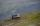Between points A, B, whose horizontal distance is 1.5 km railway line has 8promile climb. Between points B, C with horizontal distance of 900 m is climb 14promile. Calculate differences of altitudes between points A and C.
17. Rectangular trianglesThe lengths of corresponding sides of two rectangular triangles are in the ratio 2:5. At what ratio are medians relevant to hypotenuse these right triangles? At what ratio are the contents of these triangles? Smaller rectangular triangle has legs 6 and 8
18. Height differenceWhat height difference overcome if we pass road 1 km long with a pitch21 per mille?
19. RailwayRailway line had on 5.8 km segment climb 9 permille. How many meters track ascent?
20. Chimney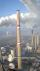Lower circumference of of the chimney is 12.57 m, top circumference is 5.655 m. The slope of the walls is 87°. Determine the height of the chimney.

Do you have an interesting mathematical word problem that you can't solve it? Submit math problem, and we can try to solve it.

We will send a solution to your e-mail address. Solved examples are also published here. Please enter the e-mail correctly and check whether you don't have a full mailbox.

Please do not submit problems from current active competitions such as Mathematical Olympiad, correspondence seminars etc...